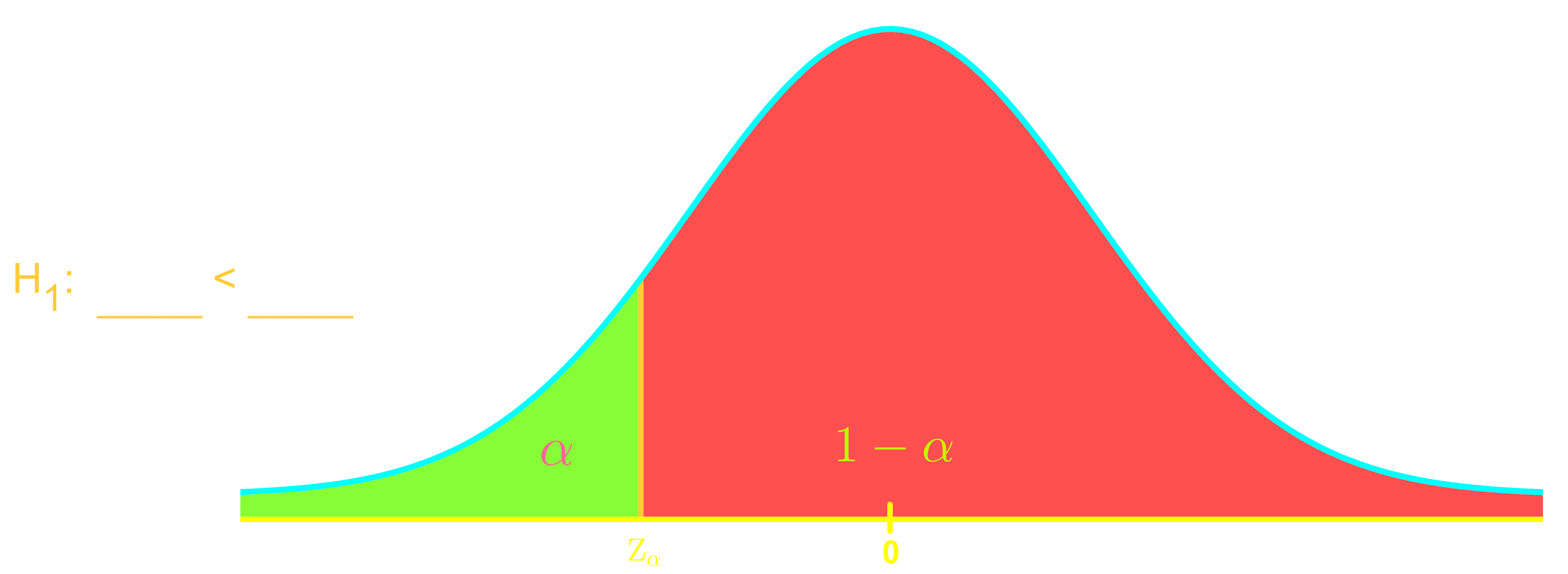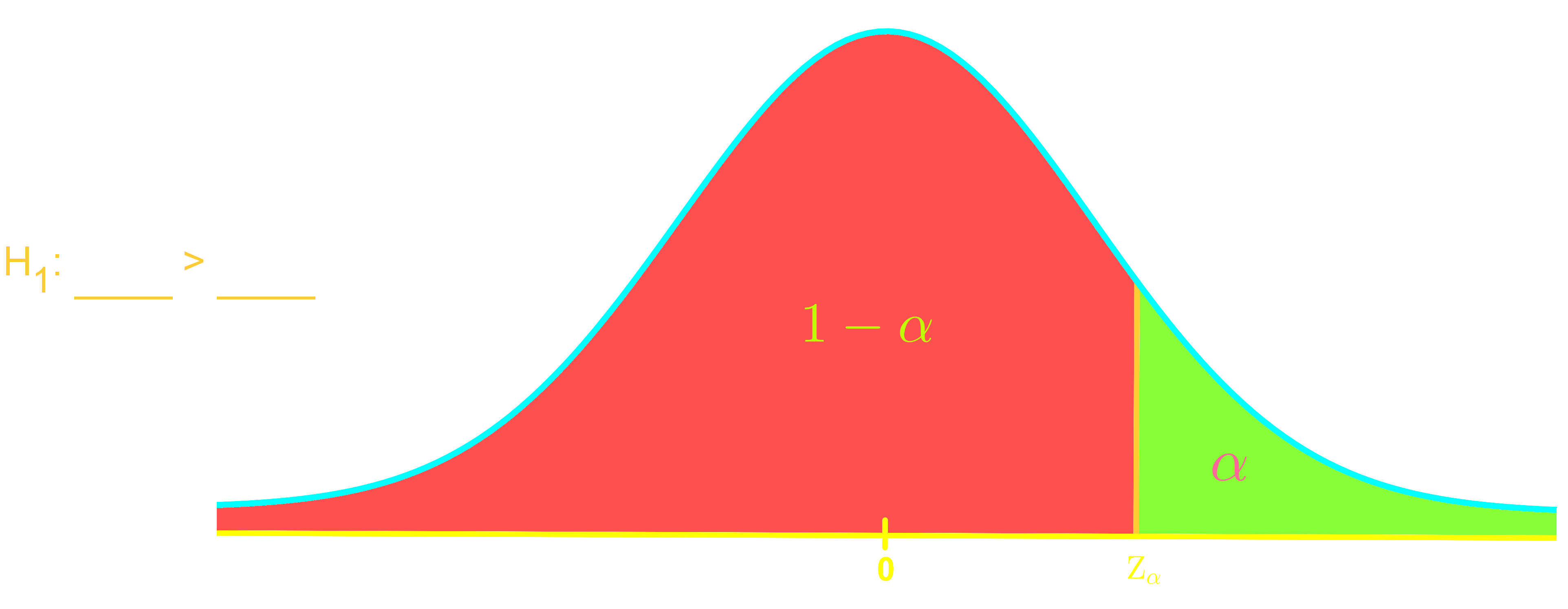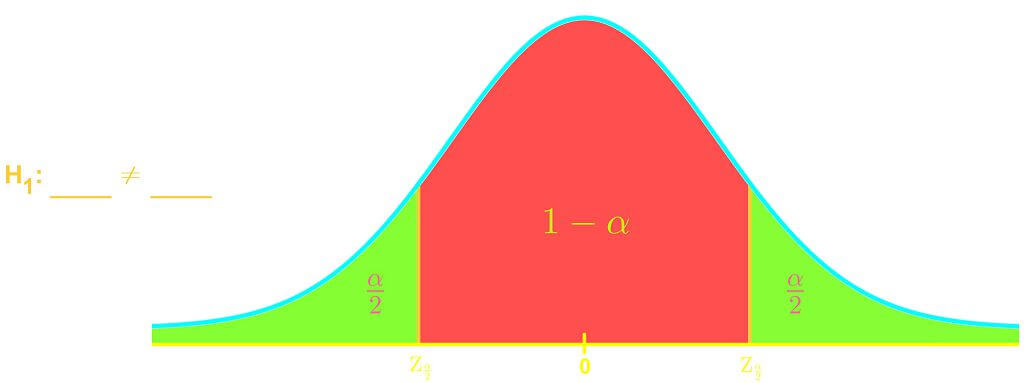# Confidence levels, significance levels and critical values

### Confidence levels, significance levels and critical values

#### Lessons

• Introduction
Using sample data, it is possible to find a Z-score relating to the claim (the test-statistic).

Significance level

 Confidence Level $(1-\alpha$) Significance Level ($\alpha$) Critical Value ($Z_{\alpha}$) 0.90 0.10 ? 0.95 0.05 ? 0.99 0.01 ?

Left Tail Test:

.Right Tail Test:

.Two tailed Test:

.• 1.
Finding the Critical Value
With a significance level of $\alpha =0.075$ what is the resulting critical value of:
a)
A right-tailed test?

b)
A left-tailed test?

c)
A two-tailed test?

• 2.
Find the critical value from the following confidence levels for a right-tailed test:
a)
90% confidence

b)
95% confidence

c)
99% confidence

• 3.
Rejecting or Failing to Reject the Null Hypothesis
The following Null Hypothesis and Alternative Hypothesis have been derived from a statement:

$H_0$:
$p \leq 0.5$

$H_1$:
$p$ > $0.5$

Using a significance level of $0.10$ (which corresponds to a critical value of $Z_\alpha=1.28$), and a Z-score of $Z=1.40$ relating to our Null Hypothesis;

Can we reject the Null Hypothesis?

• 4.
The following Null Hypothesis and Alternative Hypothesis have been derived from a statement:

$H_0$:
$\mu \geq 175 lbs$

$H_1$:
$\mu$ < $175 lbs$

Using a 95% confidence level and a Z-score of Z=-1.50 relating to our Null Hypothesis;

Can we reject the Null Hypothesis?

• 5.
The following claim is made.

"70% of Canadians own a pet".

Given that the test-statistic is Z=2.75, with a confidence level of 90% what can be said about the proportions of pet owners in Canada?